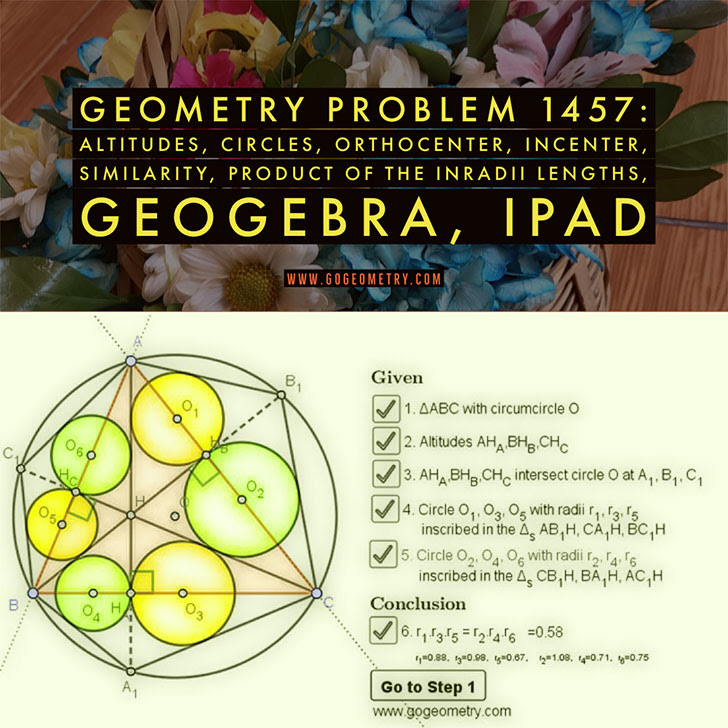## Friday, March 6, 2020

### Geometry Problem 1457: Altitudes, Circles, Similarity, Product of the Inradii Lengths

Interactive step-by-step animation using GeoGebra. Post your solution in the comment box below.
Level: Mathematics Education, High School, Honors Geometry, College.

Details: Click on the figure below.#### 1 comment:

1.Let BC1 = BH = BA1 = p, AC1 = AH = AB1 = q and CA1 = CH = CB1 = u where I have used my proof of Problem 1456

Then r1/r4 = q/p, r3/r6 =u/q and r5/r2 = p/u

Multiplying, we get r1.r3.r5 = r2.r4.r6

Sumith Peiris
Moratuwa
Sri Lanka